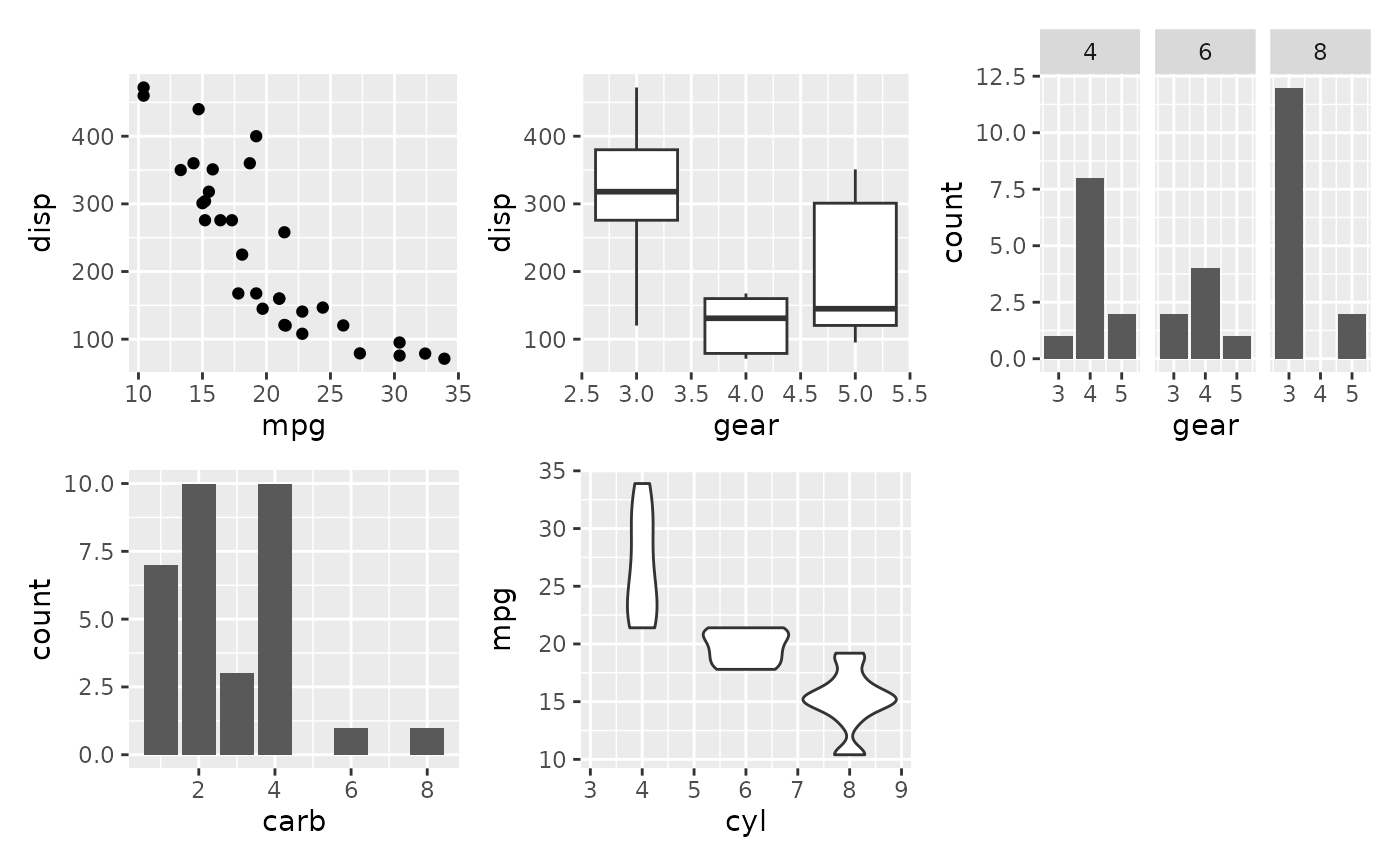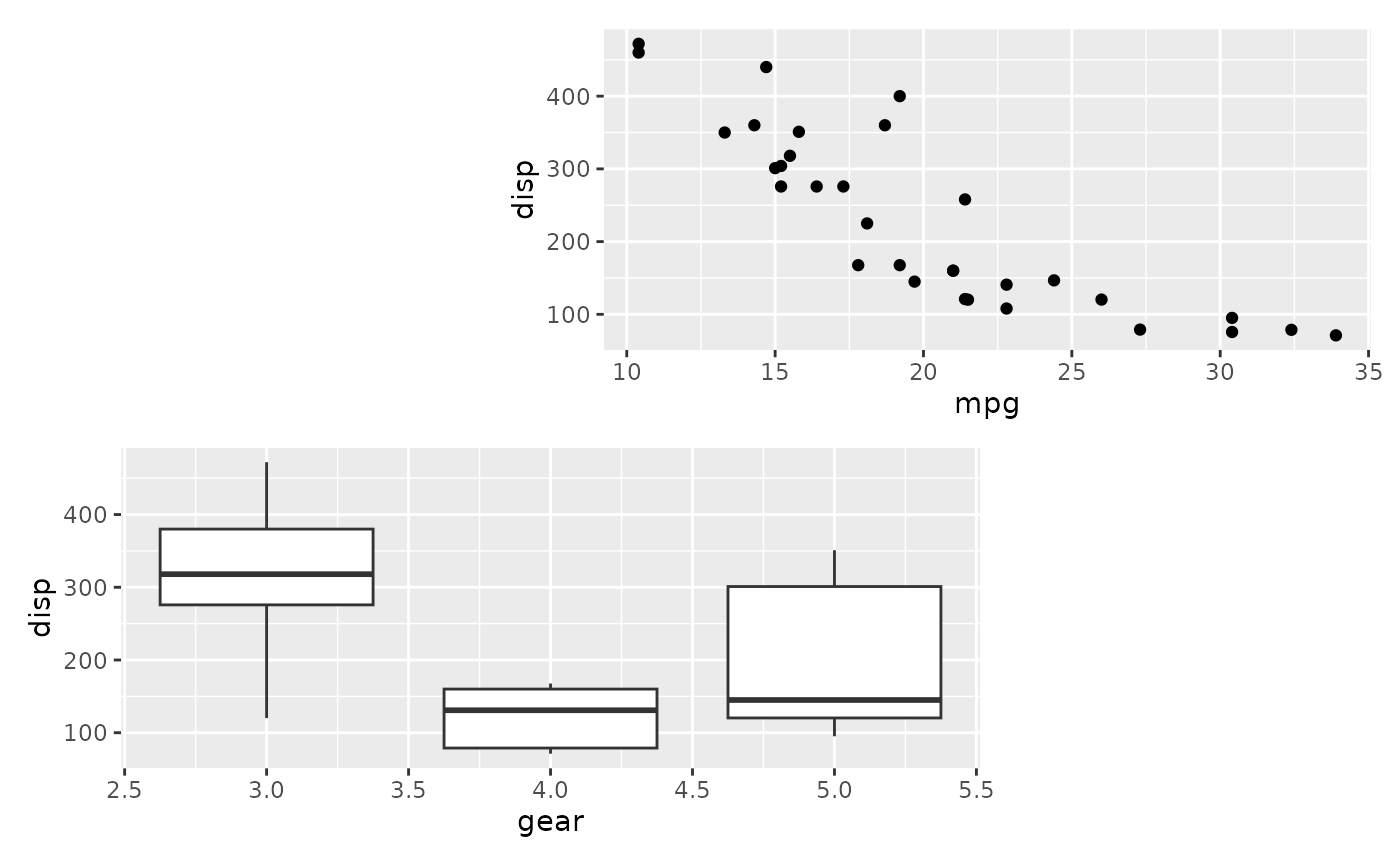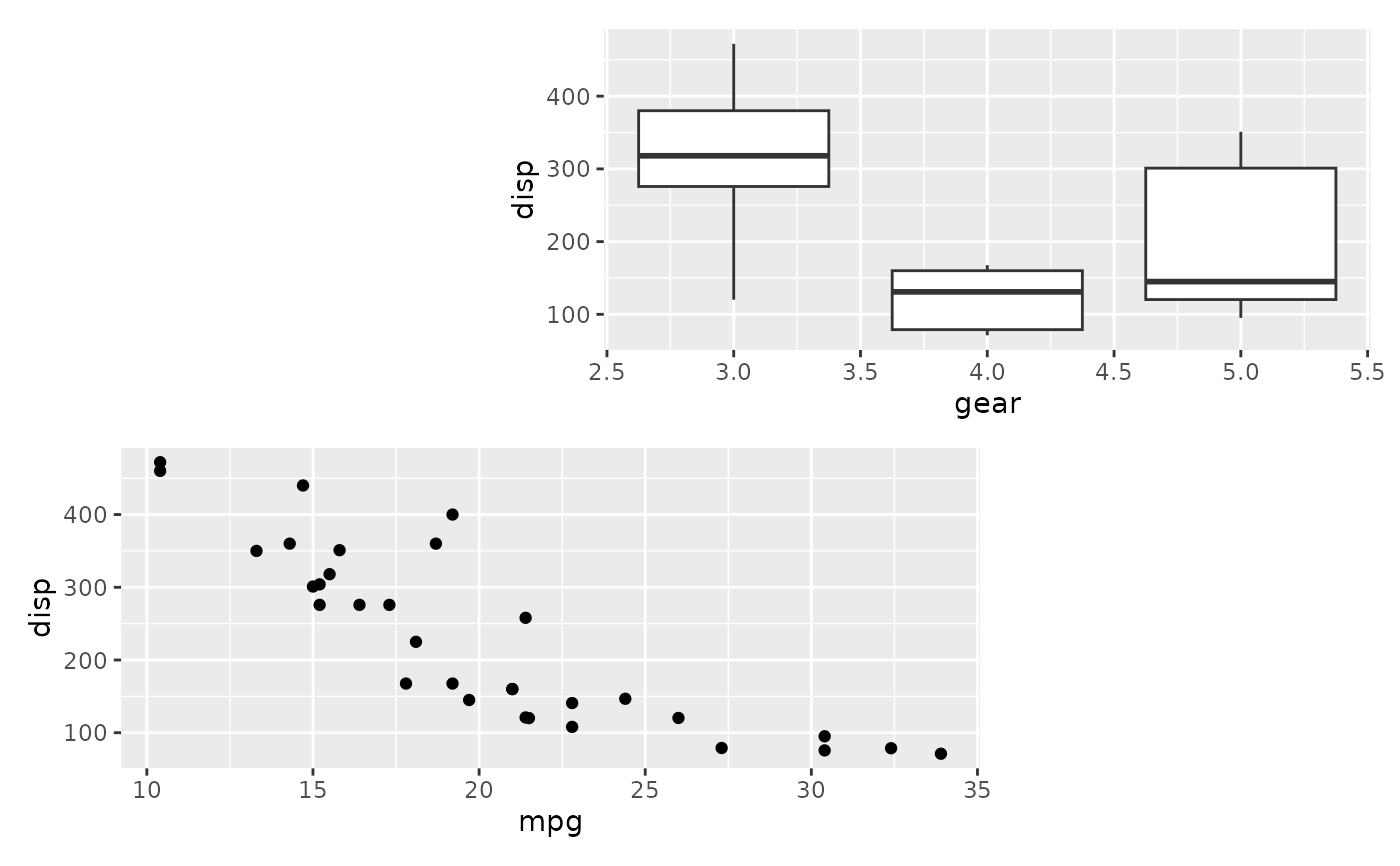While the use of + is a natural way to add plots together, it can be difficult to string together multiple plots programmatically if the number of plots is not known beforehand. wrap_plots makes it easy to take a list of plots and add them into one composition, along with layout specifications.

wrap_plots(
...,
ncol = NULL,
nrow = NULL,
byrow = NULL,
widths = NULL,
heights = NULL,
guides = NULL,
tag_level = NULL,
design = NULL
)

Arguments

... multiple ggplots or a list containing ggplot objects The dimensions of the grid to create - if both are NULL it will use the same logic as facet_wrap() to set the dimensions The dimensions of the grid to create - if both are NULL it will use the same logic as facet_wrap() to set the dimensions Analogous to byrow in matrix(). If FALSE the plots will be filled in in column-major order The relative widths and heights of each column and row in the grid. Will get repeated to match the dimensions of the grid. The relative widths and heights of each column and row in the grid. Will get repeated to match the dimensions of the grid. A string specifying how guides should be treated in the layout. 'collect' will collect guides below to the given nesting level, removing duplicates. 'keep' will stop collection at this level and let guides be placed alongside their plot. auto will allow guides to be collected if a upper level tries, but place them alongside the plot if not. If you modify default guide "position" with theme(legend.position=...) while also collecting guides you must apply that change to the overall patchwork (see example). A string ('keep' or 'new') to indicate how auto-tagging should behave. See plot_annotation(). Specification of the location of areas in the layout. Can either be specified as a text string or by concatenating calls to area() together. See the examples for further information on use.

Value

A patchwork object

Details

If design is specified as a text string and the plots are named (e.g. wrap_plots(A = p1, ...)) and all plot names are single characters represented in the design layout string, the plots will be matched to their respective area by name. Otherwise the areas will be filled out sequentially in the same manner as using the + operator. See the examples for more.

Examples

library(ggplot2) p1 <- ggplot(mtcars) + geom_point(aes(mpg, disp)) p2 <- ggplot(mtcars) + geom_boxplot(aes(gear, disp, group = gear)) p3 <- ggplot(mtcars) + geom_bar(aes(gear)) + facet_wrap(~cyl) p4 <- ggplot(mtcars) + geom_bar(aes(carb)) p5 <- ggplot(mtcars) + geom_violin(aes(cyl, mpg, group = cyl)) # Either add the plots as single arguments wrap_plots(p1, p2, p3, p4, p5)# Or add them as a list... plots <- list(p1, p2, p3, p4, p5) wrap_plots(plots)# Match plots to areas by name design <- "#BB AA#" wrap_plots(B = p1, A = p2, design = design)# Compare to not using named plot arguments wrap_plots(p1, p2, design = design)